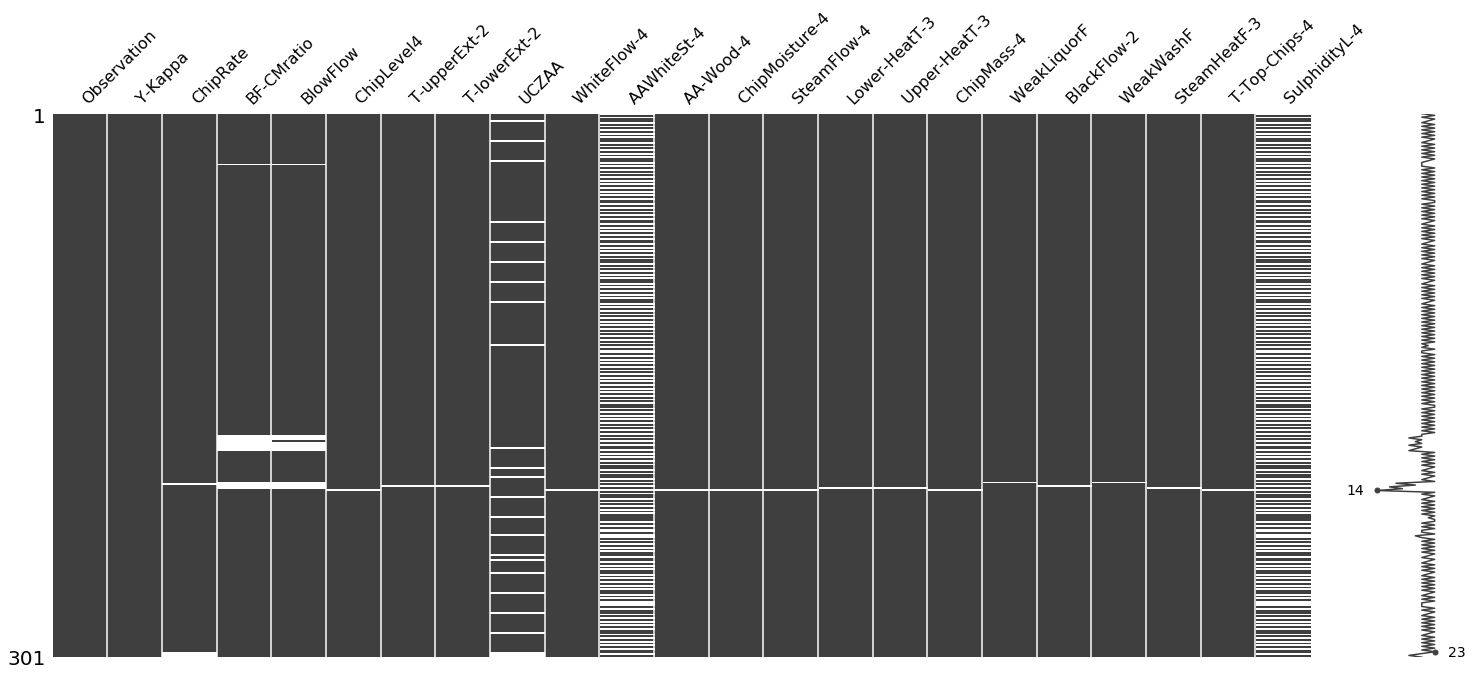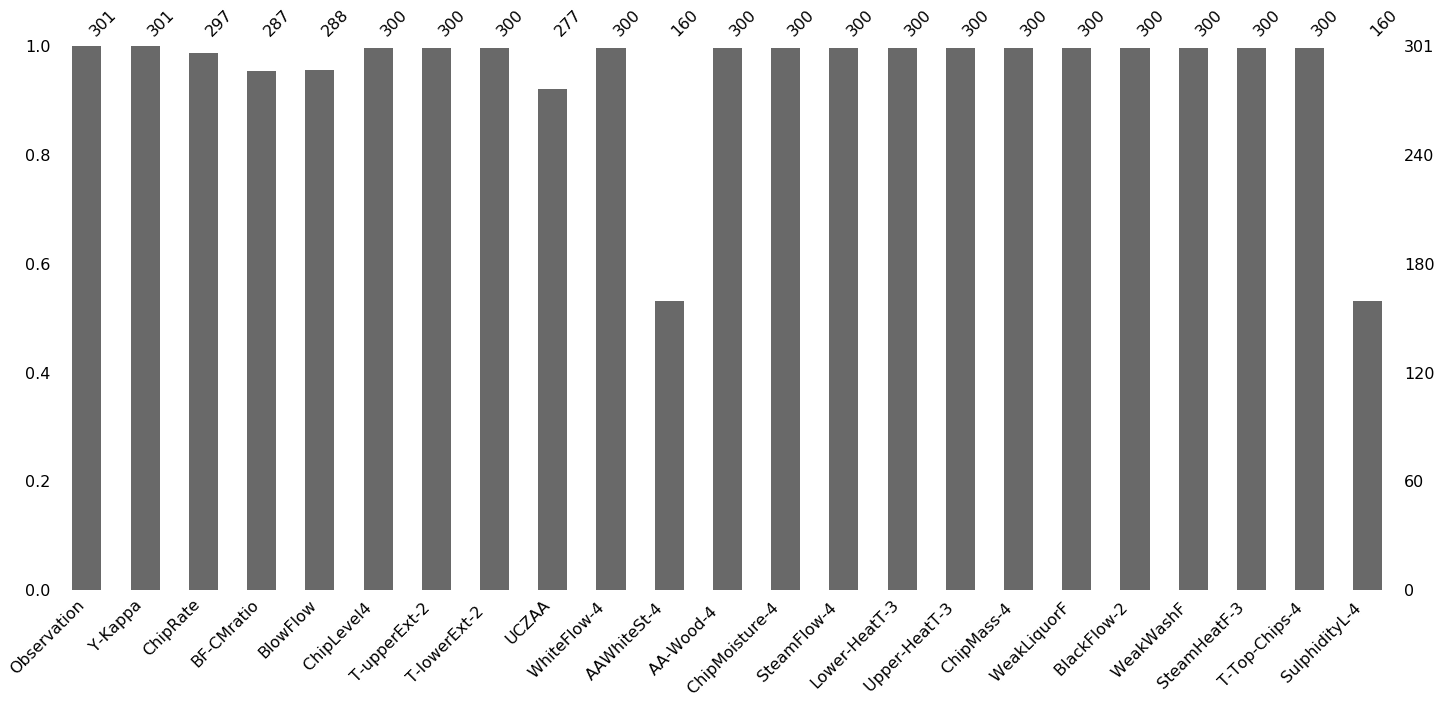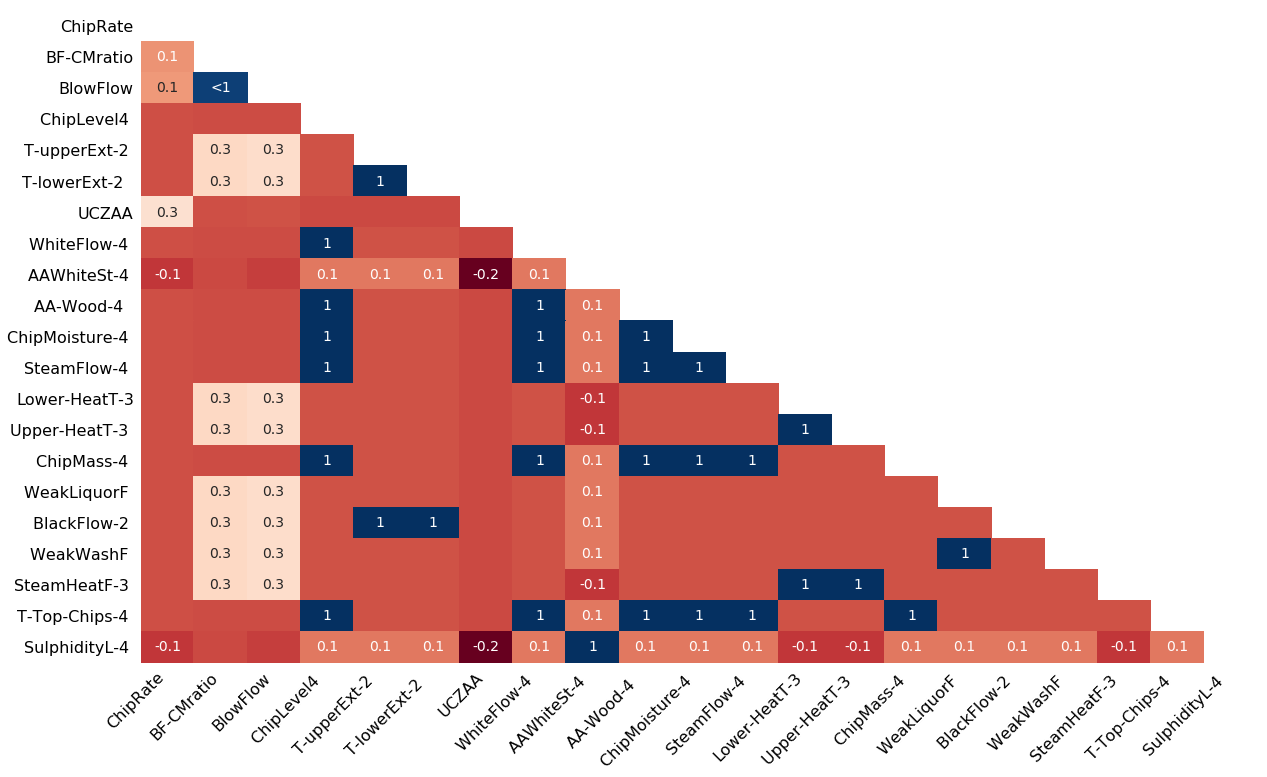# Python | Visualize missing values (NaN) values using Missingno Library

In the case of a real-world dataset, it is very common that some values in the dataset are missing. We represent these missing values as NaN (Not a Number) values. But to build a good machine learning model our dataset should be complete. That’s why we use some imputation techniques to replace the NaN values with some probable values. But before doing that we need to have a good understanding of how the NaN values are distributed in our dataset.

Missingno library offers a very nice way to visualize the distribution of NaN values. Missingno is a Python library and compatible with Pandas.

Install the library –

```pip install missingno
```

## Matrix :

Using this matrix you can very quickly find the pattern of missingness in the dataset. In our example, the columns `AAWhiteSt-4` and `SulphidityL-4` have a similar pattern of missing values while UCZAA shows a different pattern.

 `# Program to visualize missing values in dataset ` ` `  `# Importing the libraries ` `import` `pandas as pd ` `import` `missingno as msno ` ` `  `# Loading the dataset ` `df ``=` `pd.read_csv(``"kamyr-digester.csv"``) ` ` `  `# Visualize missing values as a matrix ` `msno.matrix(df) `

Output:## Bar Chart :

This bar chart gives you an idea about how many missing values are there in each column. In our example, `AAWhiteSt-4` and `SulphidityL-4` contain the most number of missing values followed by UCZAA.

 `# Program to visualize missing values in dataset ` ` `  `# Importing the libraries ` `import` `pandas as pd ` `import` `missingno as msno ` ` `  `# Loading the dataset ` `df ``=` `pd.read_csv(``"kamyr-digester.csv"``) ` ` `  `# Visualize the number of missing ` `# values as a bar chart ` `msno.bar(df) `

Output:## Heatmap :

Heatmap shows the correlation of missingness between every 2 columns. In our example, the correlation between AAWhiteSt-4 and SulphidityL-4 is 1 which means if one of them is present then the other one must be present.

A value near -1 means if one variable appears then the other variable is very likely to be missing.
A value near 0 means there is no dependence between the occurrence of missing values of two variables.
A value near 1 means if one variable appears then the other variable is very likely to be present.

 `# Program to visualize missing values in dataset ` ` `  `# Importing the libraries ` `import` `pandas as pd ` `import` `missingno as msno ` ` `  `# Loading the dataset ` `df ``=` `pd.read_csv(``"kamyr-digester.csv"``) ` ` `  ` `  `# Visualize the correlation between the number of ` `# missing values in different columns as a heatmap ` `msno.heatmap(df) `

Output:Reference : https://github.com/ResidentMario/missingno

Attention geek! Strengthen your foundations with the Python Programming Foundation Course and learn the basics.

To begin with, your interview preparations Enhance your Data Structures concepts with the Python DS Course.

My Personal Notes arrow_drop_upCheck out this Author's contributed articles.

If you like GeeksforGeeks and would like to contribute, you can also write an article using contribute.geeksforgeeks.org or mail your article to contribute@geeksforgeeks.org. See your article appearing on the GeeksforGeeks main page and help other Geeks.

Please Improve this article if you find anything incorrect by clicking on the "Improve Article" button below.